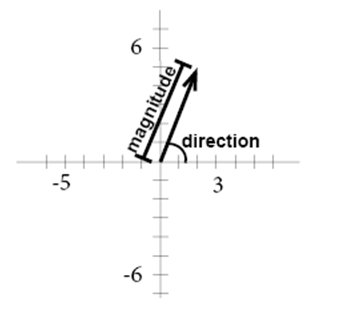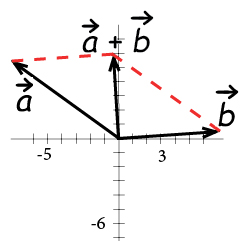# Vectors

Vectors are used to represent a quantity that has both a magnitude and a direction. The vector is normally visualized in a graph. A vector between A and B is written as

$\overrightarrow{AB}$

The vectors standard position has its starting point in origin.The component form of a vector is the ordered pair that describes the changes in the x- and y-values. In the graph above x1=0, y1=0 and x2=2, y2=5. The ordered pair that describes the changes is (x2- x1, y2- y1), in our example (2-0, 5-0) or (2,5).

Two vectors are equal if they have the same magnitude and direction. They are parallel if they have the same or opposite direction.

We can combine vectors by adding them, the sum of two vectors is called the resultant.Example

$\overrightarrow{a}=\left \langle 2,4 \right \rangle,\overrightarrow{b}\left \langle -1,6 \right \rangle$
$\overrightarrow{a}+\overrightarrow{b}=\left \langle 2+(-1),4+6 \right \rangle=\left \langle 1,10 \right \rangle$